# Microsoft Excel: Easy Data Analysis with the IF FunctionAlthough SUM is the most frequently used function in Excel, the IF function should also be in your Excel bag of workbook tricks. The IF function tests whether a condition is true or false and then performs an action such as a calculation or data entry. How often are you sorting or filtering data to manually locate Excel entries that might require additional data entry or auditing? With the IF function, you can automatically evaluate your data based on the condition or conditions that you create.

This article gives you an overview of the IF function. To see a more detailed demonstration of the various ways you can save time working with the IF function, watch my training video:

## How the IF Function Works

First, let’s look at the structure (syntax) of the IF function. As with other Excel functions, we start with =(equals). Then we add the function name followed by open parentheses, such as =IF( . The official logic and structure of an IF function is: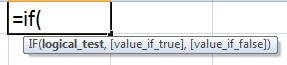=IF(what you are testing/evaluating, what to do if true, what to do if false)

## Conditions Can Be Formulas, Values, or Text

The cell or entry that is evaluated may be a formula, value, or text; the displayed result may also be a formula, value, or text answer. For example, if an amount is more than 5% over the budgeted amount, then you might display “OVER”, otherwise, show “OK.”

For instance, what if we want to calculate an analysis where, if the total is greater than or equal to \$1000, then a \$100 bonus is entered into the formula cell; otherwise, no bonus is given.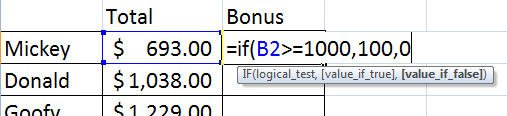The formula would look like this: =IF(B2>=1000, 100, 0) where B2 is the value being evaluated. As with other formulas, the results will update if values are changed. And, as with other formulas, this calculation can be copied down or across to calculate additional values.

The results of the IF function can also be a text entry that you may want to filter for checking data, such as =IF(B14=E14, “OK”, “AUDIT NEEDED”).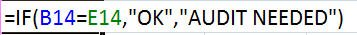In this sample function, if the two cell values are the same, the result is “OK”. Otherwise, the answer is “AUDIT NEEDED.” Notice that text entries are surrounded by quotes (to create a character string) with the formula commas outside the quotes. Note that it doesn’t matter if you add spaces after each comma.

## But Wait … There’s More! Nested Functions

Not every evaluation has only one condition limited to two different actions. Sometimes, you may have 3 or more possibilities, for instance, different calculations based on ranges or levels of values. This calls for nested/multiple IF functions. You can also nest other functions within an IF function as needed to create your logical condition. For instance, an IF function might apply a condition to the results of a SUM or AVERAGE function.

For example, the following IF function works with these options:

• If the value is less than \$25,000, multiply by 10%
• If the value is less than \$50,000 but at least \$25,000, multiply by 20%
• Otherwise (the value is greater than \$50,000), multiply by 30%

The formula would look like this: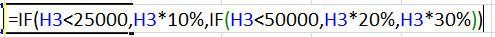NOTE: When nesting with any worksheet function, the equals sign is only required with the initial function statement, i.e. the 2nd IF in our nested formula does not need an equals sign. You can use up to 64 levels of nesting in a formula, but that’s a lot of nesting!

## Complex Functions Don’t Have to be Complicated

Ready for some more function fun? The IF function can be combined with AND, OR, NOT functions to create more detailed evaluations. These additional functions apply multiple conditions where all expressions are true (AND), only one expression needs to be true (OR), or the opposite (NOT) is true.

Looking at the breakout of Excel functions is an easy way to learn how to create your own, especially with nested functions. This next example, shown below, is designed to test: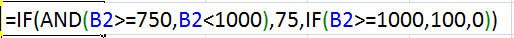• If cell B2 is between 750 and less than 1000 (AND), enter 75 in cell
• If cell B2 is greater than or equal to 1000, enter 100 in cell
• Otherwise, enter 0 in the cell (the not true/false portion)

Discover more Excel shortcuts, tips, and tricks here.

© Dawn Bjork, MCT, MOSM, CSP®, The Software Pro®
Microsoft Certified Trainer, Productivity Speaker, Certified Speaking Professional

#### Related Posts